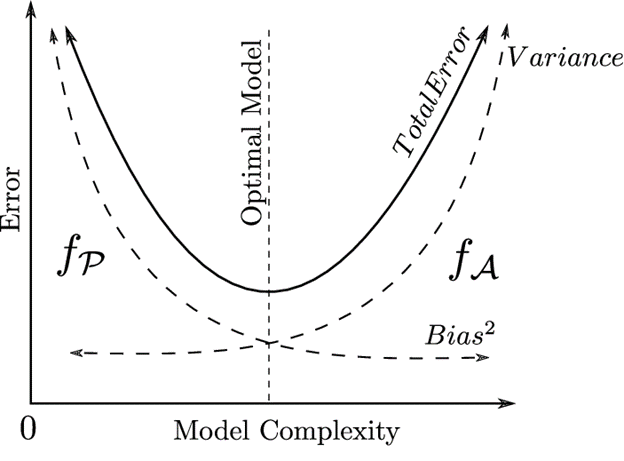SearchFor any model to perform well the error needs to be reduced. The correct balance of bias and variance is important for building any machine-learning algorithms and to create accurate results from their models. Bias and variance are used in supervised machine learning, in which an algorithm learns from training data or a sample data set of known quantities. Bias and variance are components of reducible error.

## Bias

Bias is basically how far we have predicted a value than the actual value. We can say that the bias is too high if our prediction is far off from the actual prediction. In other words, Bias means error of the training data. A high bias can cause algorithm to miss the relationship between the input and output variables as a result it oversimplifies the model and can lead to underfitting.

Let’s take an example and see what bias is ...

Let’s say we wanted to predict the height of students using the linear regressionIn the above picture we can clearly see that the straight line doesn’t have the flexibility to build the true relationship, no matter how well we fit it in the training data. The inability for a machine learning method to find the true relationship is called bias.

## Variance

When model does not perform well as it does with the train data set there is a possibility that the model has a variance. In the other words, Variance means error of the test data. Its basically telling you how scattered the predicted values are from the actual value. A high variance in the data set means that the model as trained with lot of noise and irrelevant data thus leading to overfitting.

## How does Bias and Variance affect the modelImage endtoend.ai

High Variance and High Bias: Model will be inconsistent and also inaccurate on average.

Low Variance and High Bias: Models are consistent but low on average.

High Variance and Low Bias: Somewhat accurate but inconsistent on average.

Low Variance and Low Bias: It is the ideal one where the model is consistent and accurate on average.Image from datarobot

It is a concept of finding the right balance between the Bias and the Variance so that our model isn’t overfitted nor underfitted. If the model is too simple and has a very few parameters it will suffer from high bias and low variance and on the other hand if the model has large number of parameters then it will have high variance and low bias. The trade off should result with perfectly balanced bias and variance.

## Concept of Bias and Variance Mathematically

Let the variance that we are predicting be Y and the other independent variables be X. Let us assume that there is a relationship between these two variables such that

Y=f(x)+e

Where e is the estimated error with the mean value 0 and then we make a classifier using the algorithm linear regression or Support vector machine etc... The expected squared error at a point X will be Y squared plus the variance plus the irreducible error.

Total Error = Bias²+Variance+Irreducible error

## Total error

To build any good model we need the right balance between Bias and Variance in a way that it minimizes total error.Image ResearchGate

Total Error = Bias²+Variance+Irreducible error

A perfect balance of Bias and Variance will never underfit or overfit. It is very essential for any data scientist to understand the concept of bias and variance to reduce the errors and build accurate models.